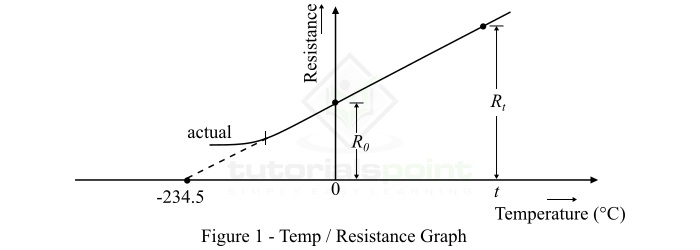# Factors Affecting the Resistance of a Conductor

In this article, we will discuss the factors affecting the resistance of a conductor and the change in the resistance of a substance with the variation in temperature. Let’s begin with a basic introduction of electrical resistance.

Electrical resistance is defined as the measure of opposition that a material offers in the path of electric current. The electrical resistance is denoted by the symbol R and is measured in Ohms (Ω).

The electrical resistance of a conductor is given by the following empirical formula,

$$\mathrm{R=\frac{\rho l}{a}\: \: \cdot \cdot \cdot \left ( 1 \right )}$$

Where, ρ (rho) is a constant called resistivity or specific resistance of the material of the conductor, l is the length of the conductor, and a is the area of the cross-section of the conductor.

## Factors Affecting the Resistance

From equation (1), it is clear that the electrical resistance of a conductor −

• Is directly proportional to the length (l) of the conductor.
• Is inversely proportional to the cross-sectional area (a) of the conductor.
• Depends upon the nature of the material (ρ).
• Changes with the change in temperature.

## Resistance Variation with Temperature

As discussed in the above section, the resistance of a material varies with the change in its temperature. In this section, we will understand the variation of resistance with temperature for different types of engineering materials such as metals, semiconductors, electrolytes, metal alloys, and semiconductors.

From elementary physics, we know that electrical resistance is the opposition in the flow of electric charge through the material due to collision with positive atoms of the material. This opposing property of materials is referred to as resistivity. The resistivity of materials depends upon the nature of the material and its temperature. This is due to the fact that the temperature influences the number of electric charges (free electrons) in the material and hence affects its resistivity or resistance.

The resistance of different materials varies differently with the temperature. Because, in some materials, the rise in temperature increases the number of electrons or electric charge, while in some other materials, the increase in temperature reduces the number of free electrons. Now, let us discuss the variation of resistance with the temperature for different types of materials individually −

## Change in Resistance of Metals with Temperature

In the case of metals, the electrical resistance is mainly due to collisions of free electrons with the positive atoms of the metal. Hence, when the temperature of metals increases, it increases the velocity of the electrons. Consequently, the number of collisions increased per unit of time. In this way, the resistance of metals increases with the increase in temperature.

But, the variation in the resistance of metals is regular for a normal range of temperatures, hence the curve of their temperature-resistance graph is a straight line as shown in Figure-1.Since the electrical resistance of metals increases with the increase in their temperature. Therefore, metals have a positive temperature coefficient of resistance.

## Change in Resistance of Semiconductors and Insulators

Both semiconductors and insulators are bad conductors of electric current (i.e. electric charge). Hence, they offer very high resistance to the flow of electric charge at normal temperatures. This is because, at normal temperature, the number of free electrons in semiconductors and insulator are negligible. But, when we increase the temperature, electrons in their atoms gain energy and become free by breaking the chemical bonds. Thus, the resistance of semiconductors and insulators decreases with the rise in temperature.

Since the resistance of semiconductors and insulators decreases with the increase in temperature. Therefore, they have a negative temperature coefficient of resistance.

## Change in Resistance of Electrolytes

The conducting mediums that conduct electricity by the movement of ions, but not by the movement of free electrons, are called electrolytes. Electrolytes are usually solutions of salts. The number of ions in an electrolyte is influenced by the temperature, i.e. ions in an electrolyte increase with the rise in temperature. Therefore, the resistance of electrolytes also decreases with the increase in temperature.

Since the resistance of electrolytes decreases with the rise in temperature, they also have a negative temperature coefficient of resistance.

## Change in Resistance of Alloys

Alloys are the mixtures of several different metals, hence an alloy has combined characteristics of its constituent metals. Since the resistance of metals increases with the rise in temperature. Consequently, the resistance of alloys also increases with the increase in temperature, but unlike metals, this increase is very small and irregular. For some alloys like constantan, manganin, etc., the variation in resistance is practically negligible over a wide range of temperatures.

Since the resistance of alloys also increases with the rise in temperature, thus they have a positive temperature coefficient of resistance.

## Conclusion

The resistance variation of a material with temperature can be concluded as follows: "When the temperature of a material increases, the vibrations of the molecules increase accordingly. These vibrations restrict the movement of free electrons through the material. This results in the increase in resistance of the material."

In the case of metals, with the increase in temperature, there is no increase in the number of free electrons. The net effect of the temperature rise is to increase in the resistance of the metal due to increased molecular vibrations.

But, in the case of semiconductors and insulators, the increase in temperature creates more free electrons within the material. Thus, the electrical resistance of such materials decreases with the rise in temperature.